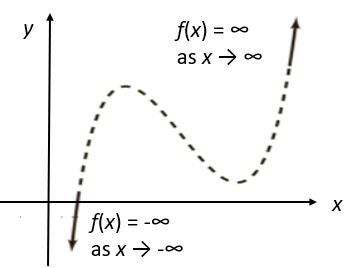# Coefficient, Leading Coefficient: Definition, Test

## Definition

Coefficients are numbers or letters used to multiply a variable.  A variable is defined as a symbol (like x or y) that can be used to represent any number.  In a function, the coefficient is located next to and in front of the variable.  Single numbers, variables or the product of a number and a variable are called terms.

## Coefficient Example

3x – 1xy + 2.3 + y

In the function above the first two coefficients are 3 and 1.  Notice that 3 is next to and in front of variable x, while 1 is next to and in front of xy

The third coefficient is 2.3.  This is called a constant coefficient since its value will not change since it is not being multiplied by a variable.  Simply defined, a constant is a term without a variable.

The fourth term (y) doesn’t have a coefficient.  In these cases, the coefficient is considered to be 1 since multiplying by 1 wouldn’t change the term.

## Like Terms

Like terms are terms that have the same variable raised to the same power.  The function above doesn’t have any like terms, since the terms are 3x, 1xy, 2.3 and y and they all have different variables.

## Example of Like Terms

2xy2 + 3xy2 – 5xy2

Notice that the coefficients (2, 3 and 5) are all different values.  However, the function contains like terms since the variable (xy) for each term are raised to the second power.

Above we defined coefficients as being either numbers or letters.  You may come across a function with no numerical value in the coefficient spot.  Just treat the letter located in front of and next to the variable as the coefficient.  For example:

ax + bx + c

In the function above a and b are coefficients while x is a variable.  The third term (c) does not have a coefficient so the coefficient is considered to be 1.

## Examples of Coefficients

5 x4+ 567 x2 + 24,
The coefficients are:

• 5, which acts on the x4 term.
• 567, which acts on the x2.
• 24.

What about 24? It acts on a special, invisible term; the x0 term. Since any number to the 0th power is always 1, it’s normally condensed down to 1—or, when written with the coefficient, skipped altogether. The coefficient of the x0 is the constant coefficient.

x5 + 21 x 3 + 6 x 5
The coefficients are:

• 1,
• 21,
• 6.

The fact that no number is written in front of x5 tells us immediately that the coefficient is the identity coefficient, the one number that leaves identical whatever it multiplies.

24 x 8 + 56 7 + 22
The coefficients are:

• 24,
• 56,
• 22.

The leading coefficient is the coefficient of the highest-order term; the term in which our variable is raised to the highest power. In this case, that is x 8, so the leading coefficient is 24.

## Nonconstant Coefficients

A coefficient can’t include the variables it acts upon, but it isn’t always a constant either. When it’s not a constant, the variables it includes are called parameters. In the equation y x4 + 4y x2 + 3 x2 + 4 x the coefficients are y, 4y + 3, and 4.

## What is a Leading Coefficient?

In a polynomial function, the leading coefficient (LC) is in the term with the highest power of x (called the leading term). As polynomials are usually written in decreasing order of powers of x, the LC will be the first coefficient in the first term.

The leading coefficient test uses the sign of the leading coefficient (positive or negative), along with the degree to tell you something about the end behavior of graphs of polynomial functions.

You have four options:

## 1. Odd Degree, Positive Leading Coefficient

The graph drops to the left and rises to the right:## 2. Odd Degree, Negative LC

The graph rises on the left and drops to the right:## 3. Even Degree, Positive Leading Coefficient

The graph rises on both ends:## 4. Even Degree, Negative LC

The graph drops on both ends:Note that the test only tells you what’s happening at the ends of the graphs; It says nothing about what’s going on in the middle (which is largely determined by the polynomial’s degree). The dashed line in the examples indicate that the shape there is not determined by this particular test.

## ExampleThe above graph shows two functions (graphed with Desmos.com):

1. -3x3 + 4x = negative LC, odd degree. The graph rises on the left and drops to the right.
2. 4x2 + 4 = positive LC, even degree. The graph rises on both sides.

## Other Uses (Specialized Coefficients)

The term “coefficient” is used in dozens of different ways in other fields. For example, in statistics, correlation coefficients tell us whether two sets of data are connected. They are also measures of reliability (e.g. two judges agreeing on a certain ranking) and agreement (the stability or consistency of test scores).

## Correlation coefficients

These tell us whether two sets of data are connected:

## Coefficients that measure agreement

Coefficients that measure agreement (e.g. two judges agreeing on a certain ranking) include:

## References

Crossland, T. Polynomial Functions Terminology. Retrieved Feb 17, 2023 from: http://www.pstcc.edu/facstaff/tlcrossl/PA002_3%20polynomial%20functions.pdf
Gonick, L. The Cartoon Guide to Calculus.
Larson, R. (2011). Calculus with Precalculus. Cengage Learning.
University of Arizona. (2006). Polynomial Functions. Retrieved July 10, 2020 from: http://www.biology.arizona.edu/biomath/tutorials/polynomial/Polynomialbasics.html

CITE THIS AS:
Stephanie Glen. "Coefficient, Leading Coefficient: Definition, Test" From StatisticsHowTo.com: Elementary Statistics for the rest of us! https://www.statisticshowto.com/leading-coefficient-definition-test/